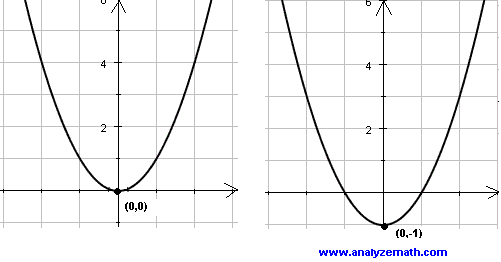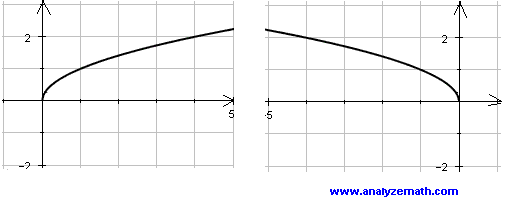# Graphing by Translation, Scaling and Reflection

A step by step tutorial on the properties of transformations such as vertical and horizontal translation (or shift) , scaling and reflections on x-axis and y-axis of graphs of functions is presented.

## Translation / Shifting Horizontally

Take any function f(x) and change x to x + c, the graph of f(x + c) will be the graph of f(x) shifted horizontally c units.

If c is negative, then the graph is shifted to the right. The example of the graph of f(x) = x2 and g(x) = (x - 2)2 are shown below and it is easily seen that the graph of (x - 2)2 is that of x2 shifted 2 units to the right.

 f(x) = x2 g(x) = (x - 2)2If c is positive, then the graph is shifted to the left. The example of the graph of f(x) = √(x) and g(x) = √(x + 2) are shown below and it is easily seen that the graph of √(x + 2) is that of √(x) shifted 2 units to the left.

 f(x) = √x g(x) = √(x + 2)If we add a constant c to f(x), the graph of f(x) + c will be the graph of f(x) translated (or shifted) vertically. If c is positive, the graph is translated up as shown in the graph below.

## Translation / Shifting Vertically

 f(x) = x2 g(x) = x2 + 2If c is negative, the graph is translated down as shown in the graph below.

 f(x) = x2 g(x) = x2 - 1## Reflection on y-axis

Take any function f(x) and change x to - x, the graph of f(- x) will be the graph of f(x) reflected on the y axis.
 f(x) = √(x) g(x) = √(- x)## Reflection on x-axis

Take any function f(x) and it to - f(x), the graph of - f(x) will be the graph of f(x) reflected on the x axis.
 f(x) = |x| g(x) = - |x|## Scaling Vertically

Take any function f(x), the graph of k f(x) (with k > 0) will be the graph of f(x) expanded vertically if k is greater than 1 and compressed vertically if k is less than 1.
Graph in blue is that of f(x) = |x| and the graph in red is that of g(x) = 2 |x|## More References and Links to Graphing

Graphing Functions.